# Logarithmic Scale: Definition & Overview

Lesson Transcript
Instructor: Scott van Tonningen

Scott has a Ph.D. in electrical engineering and has taught a variety of college-level engineering, math and science courses.

A logarithmic scale is a useful way to represent information on a graph, especially when there are exponential changes in the magnitude of the numbers. Learn more, look at several examples and take a quiz to test your knowledge.

## What Is a Logarithmic Scale?

Have you ever accidentally left some raw chicken out on the kitchen counter for four hours? If you have, then you knew that you had to throw it away, because there are often dangerous bacteria already in raw chicken, and at room temperature, they can multiply quickly. Under ideal conditions some bacteria cells can divide in only 30 minutes, and those cells divide again after another 30 minutes. If this process starts with just one cell, the exponential growth of the bacteria can yield almost 17 million cells in just 12 hours! The following chart illustrates this growth: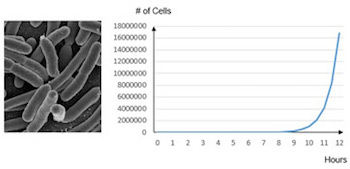This is a good chart, but it has a problem. Each axis in the graph uses a linear scale, so while the information is being represented accurately, it's really hard to tell what's happening with bacteria growth until about the 9-hour point. We need a way to rescale one of the axes so that all of the information can be used. It is for this reason that logarithmic scales were developed.

Recall that a logarithm is just the power to which a base is raised to equal a particular number. In the following example, the base is 10 and the logarithm is 1.7: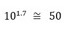The definition of a logarithmic scale is one in which the units on the axis are powers, or logarithms, of a base number, and it is typically used when the increase or decrease in value on that axis is exponential. Most often, the base used is 10. If only one axis uses a logarithmic scale, then it is defined as a semi-log chart. If both axes use a logarithmic scale, then it is defined as a log-log chart. A logarithmically scaled axis can never represent zero or a negative number, because there is no power to which you can raise a base number to get numbers less than or equal to zero. We can have logarithms that are equal to zero, but remember that they represent the number 1; negative logarithms are also used, as we will see in an upcoming example.

In order to get more insight from the bacterial growth chart, we can change one of the linearly scaled axes to a logarithmic scale, thus creating a semi-log plot. Let's reformat the y-axis using powers of 10 to represent the cell growth numbers: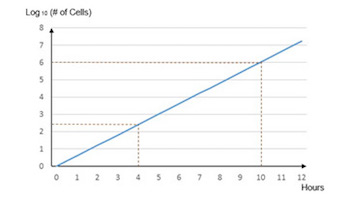What a difference! Now, instead of a linear y-axis, each major unit is a power of 10. The bottommost logarithm is zero, and 100 represents the number 1. The next line up is 101 or 10. The next is 102 = 100 and so forth. By doing this, we have now created a graph that is a straight line, and it is easier to pull information from the chart. For example, when you left the chicken out, you can see how many bacteria would have grown from a single cell. The log value at 4 hours is about 2.4. 10 raised to the 2.4 power is 251 cells. At 10 hours, the log value is about 6 and 106 is 1,000,000 cells.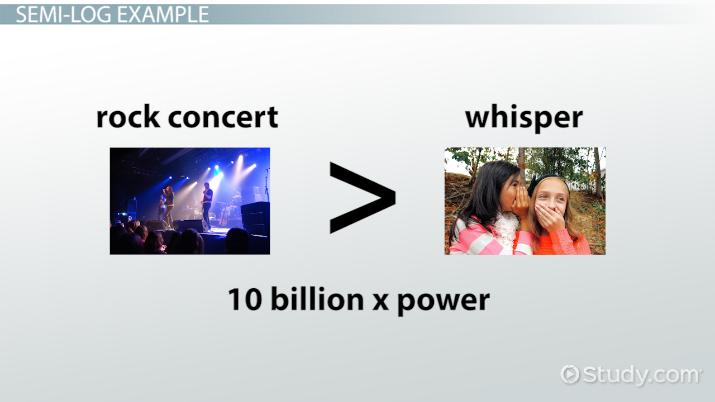An error occurred trying to load this video.

Try refreshing the page, or contact customer support.

Coming up next: What is Pangaea? - Theory & Definition

### You're on a roll. Keep up the good work!

Replay
Your next lesson will play in 10 seconds
• 0:00 What Is a Logarithmic Scale?
• 3:05 Semi-Log Example
• 4:36 A Log-Log Example
• 7:01 Lesson Summary
Save Save

Want to watch this again later?

Timeline
Autoplay
Autoplay
Speed Speed

## Semi-Log Example

Let's look at another semi-log example:

What's the loudest sound you've ever heard? Maybe it was a rock concert or a clap of thunder. And how about the quietest sound? A whisper? Common audio power measurements put the rock concert at 10 billion times the power output of the whisper! And yet, the whisper is measured as having 100 times the power of the weakest recorded sound. The chart below shows the audio power output of some familiar sounds multiplied by the power of the weakest sound (which has a power of 1):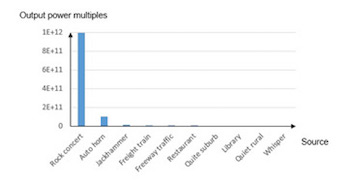Once again, we have an accurate chart, but many of the noise sources have comparatively low power associated with them, so they appear to be insignificant. Let's again create a semi-log chart by changing the y-axis to base 10 logarithms. This gives us a new chart that can provide more insight: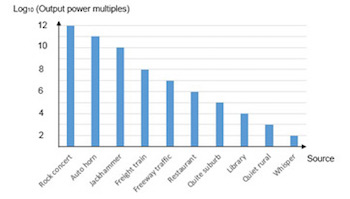Now we can get a better idea of the power level attributed to each noise source. Freeway traffic, for example, has a logarithm of 7. 107 is 10,000,000. So, freeway traffic has an audio power that is 10 million times greater than the weakest recorded sound. These charts also provide a convenient way to do comparisons. Suppose we wanted to know how much louder a jackhammer is than a library. The jackhammer has a logarithm of 10, and the library is 4. Exponents subtract, so 10 - 4 = 6 and we can raise 10 to the 6th power to see how much louder the jackhammer is (one million times) than the library.

## A Log-Log Example

Now let's look at a log-log example:

To unlock this lesson you must be a Study.com Member.

### Register to view this lesson

Are you a student or a teacher?